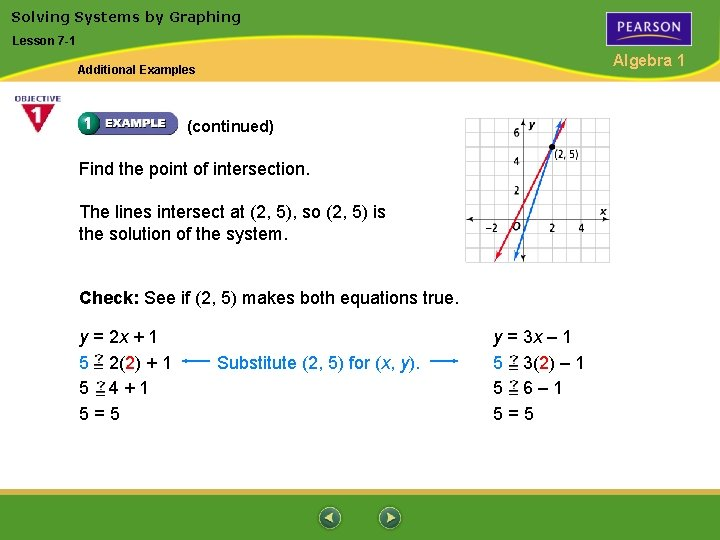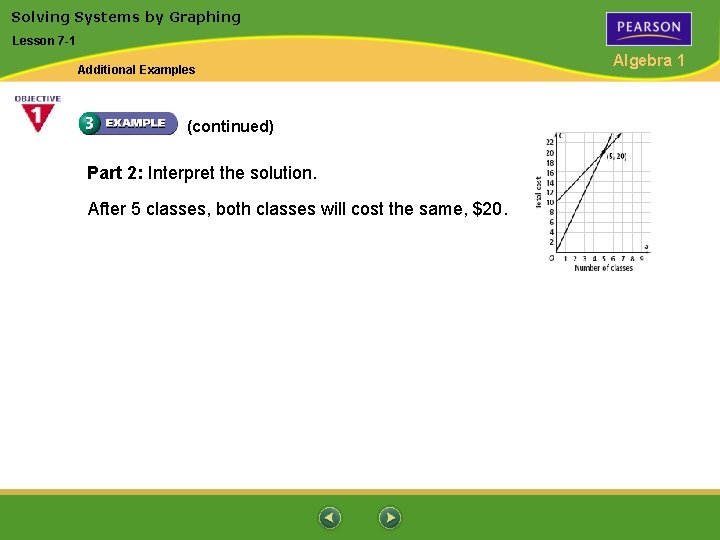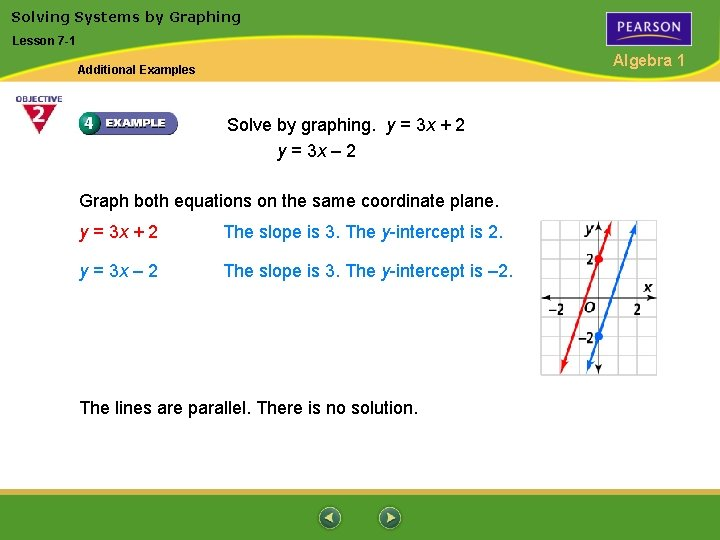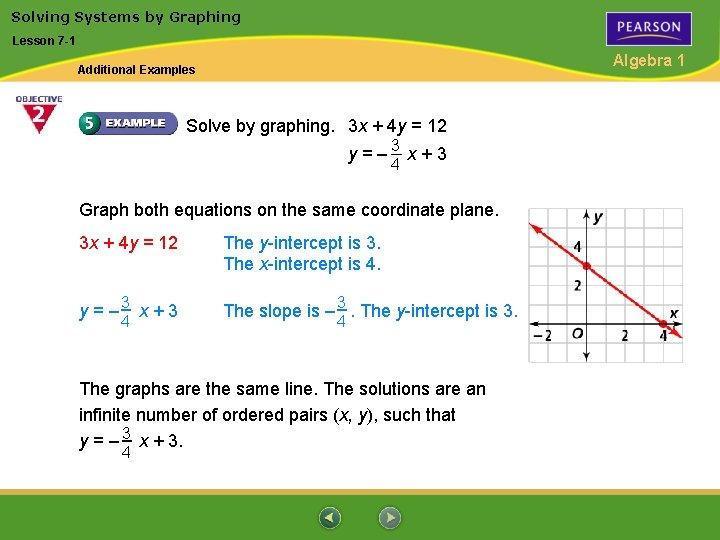# Solving Systems by Graphing Lesson 7 1 Algebra

• Slides: 8Solving Systems by Graphing Lesson 7 -1 Algebra 1 Additional Examples Solve by graphing. Check your solutions. y = 2 x + 1 y = 3 x – 1 Graph both equations on the same coordinate plane. y = 2 x + 1 y = 3 x – 1 The slope is 2. The y-intercept is 1. The slope is 3. The y-intercept is – 1.Solving Systems by Graphing Lesson 7 -1 Algebra 1 Additional Examples (continued) Find the point of intersection. The lines intersect at (2, 5), so (2, 5) is the solution of the system. Check: See if (2, 5) makes both equations true. y = 2 x + 1 5 2(2) + 1 5 4+1 5=5 Substitute (2, 5) for (x, y). y = 3 x – 1 5 3(2) – 1 5 6– 1 5=5Solving Systems by Graphing Lesson 7 -1 Algebra 1 Additional Examples Suppose you plan to start taking an aerobics class. Non-members pay \$4 per class while members pay \$10 a month plus an additional \$2 per class. Which system of equations models the cost as a function of classes? A C(a) = 4 a C(a) = 10 + 2 a B C(a) = a + 4 C(a) = 10 + 2 a C C(a) = 4 a C(a) = 10 a + 2 D C(a) = a + 4 C(a) = 10 a + 2 Define: Let a = number of classes. Let C(a) = total cost of the classes.Solving Systems by Graphing Lesson 7 -1 Algebra 1 Additional Examples (continued) Relate: cost is Write: member C(a) = 10 + 2 a = 0 + 4 a non-member C(a) The system is C(a) = 4 a C(a) = 10 + 2 a The answer is A. membership fee plus cost of classes attendedSolving Systems by Graphing Lesson 7 -1 Algebra 1 Additional Examples The system below models the cost of taking an aerobics class as a function of the number of classes. Find the solution of the system by graphing. What does the solution mean in terms of the situation? Part 1: Find the solutions by graphing. C(a) = 2 a + 10 The slope is 2. The intercept on the vertical axis is 10. C(a) = 4 a The slope is 4. The intercept on the vertical axis is 0. Graph the equations. C(a) = 2 a + 10 C(a) = 4 a The lines intersect at (5, 20).Solving Systems by Graphing Lesson 7 -1 Additional Examples (continued) Part 2: Interpret the solution. After 5 classes, both classes will cost the same, \$20. Algebra 1Solving Systems by Graphing Lesson 7 -1 Algebra 1 Additional Examples Solve by graphing. y = 3 x + 2 y = 3 x – 2 Graph both equations on the same coordinate plane. y = 3 x + 2 The slope is 3. The y-intercept is 2. y = 3 x – 2 The slope is 3. The y-intercept is – 2. The lines are parallel. There is no solution.Solving Systems by Graphing Lesson 7 -1 Algebra 1 Additional Examples Solve by graphing. 3 x + 4 y = 12 y=– 3 x+3 4 Graph both equations on the same coordinate plane. 3 x + 4 y = 12 The y-intercept is 3. The x-intercept is 4. y = – 3 x + 3 The slope is – 3. The y-intercept is 3. 4 4 The graphs are the same line. The solutions are an infinite number of ordered pairs (x, y), such that y = – 3 x + 3. 4